# Group Theory: Theory

Symmetry can help resolve many chemistry problems and usually the first step is to determine the symmetry. If we know how to determine the symmetry of small molecules, we can determine symmetry of other targets which we are interested in. Therefore, this module will introduce basic concepts of group theory and after reading this module, you will know how to determine the symmetries of small molecules.

## Introduction

Symmetry is very important in chemistry researches and group theory is the tool that is used to determine symmetry. Usually, it is not only the symmetry of molecule but also the symmetries of some local atoms, molecular orbitals, rotations and vibrations of bonds, etc. that are important. For example, if the symmetries of molecular orbital wave functions are known, we can find out information about the binding. Also, by the selection rules that are associated with symmetries, we can explain whether the transition is forbidden or not and also we can predict and interpret the bands we can observe in Infrared or Raman spectrum.

Symmetry operations and symmetry elements are two basic and important concepts in group theory. When we perform an operation to a molecule, if we cannot tell any difference before and after we do the operation, we call this operation a symmetry operation. This means that the molecule seems unchanged before and after a symmetry operation. As Cotton defines it in his book, when we do a symmetry operation to a molecule, every points of the molecule will be in an equivalent position.

## Symmetry Elements

For different molecules, there are different kinds of symmetry operations we can perform. To finish a symmetry operation, we may rotate a molecule on a line as an axis, reflect it on a mirror plane, or invert it through a point located in the center. These lines, planes, or points are called symmetry elements. There may be more then one symmetry operations associated with a particular symmetry

#### Identity E

The molecule does not move and all atoms of the molecule stay at the same place when we apply an identity operation, E, on it. All molecules have the identity operation. Identity operation can also be a combination of different operations when the molecule returns to its original position after these operations are performed.1 This will be demonstrated later.

#### Proper Rotations and Cn axisCn generates n operations, whose symbols are Cn, Cn2, Cn3, Cn4,…, E (=Cnn). However, we usually write them in another way. Table 1.2 shows the way we write the 6 operations generated by proper rotation C6. From this table, we can see that the symbols of the 6 rotations generated by C6 are C6, C3, C2, C32, C65, E.One molecule can have many proper axes and the one with the largest n is called principle axis.

Table 1.2 C6 axis and operations it generates#### Reflection and mirror plane σ

Take NH3 for an example. There are 3 mirror planes in molecule NH3. When we do a reflection through a mirror plane, molecule NH3 dose not change (Figure 1.2).Figure 1.2 A mirror plane of NH3.

There are three different kinds of mirror plane, $$\sigma_v$$, $$\sigma_h$$, and $$\sigma_d$$. The mirror plane that contains the principle axis is called $$\sigma_v$$.The mirror plane that perpendicular to the principle axis is called σh.2 Figure 1.3 shows $$\sigma_v$$, $$\sigma_h$$, and $$\sigma_d$$ in $$PtCl_4^{2-}$$.Figure 1.3 $$\sigma_v$$, $$\sigma_h$$, and $$\sigma_d$$ of PtCl42-.This picture is drawn by ACD Labs 11.0.

When mirror plane is operated n times, we have1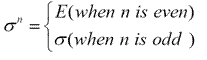#### Inversion and inversion center iIn a molecule, if we can find a point, on the straight line through which we can find a pair of same atoms on both side of this point, we call this molecule has an inversion center. The inversion center, i, is not necessarily on an atom of the molecule.1Figure 1.4 shows the inversion center of $$C_2H_4Cl_2$$.When inversion is operated n times, we have1Figure 1.4 Inversion center of C2H4Cl2.

#### Improper Rotations and Sn axis

Improper rotation is a combination of two operations, proper rotation Cn and reflection ?. Figure 1.5 shows the improper rotation operation in CH4.

Table 1.3 and table 1.4 show the operations generated by S6 and S5 axes separately. The 6 operations generated by S6 axis are S6, C3, i, C32, S65 and E. And the 10 operations generated by S5axis are S5, C52, S53, C54, ?, C5, S57, C53, S59 and E.1Since S1= $$\sigma_h$$ and S2hC2=i, the order of improper rotation, n, must always be larger than 2. And generally, when n is even, there are n operations {Sn1, Sn2, ..., Snn}, while when n is odd, there are 2n operations {Sn1, Sn2, ..., Sn2n}. And we have1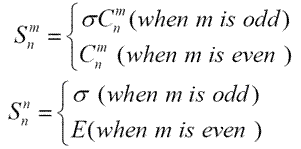Table 1.3 S6 axis and operations it generates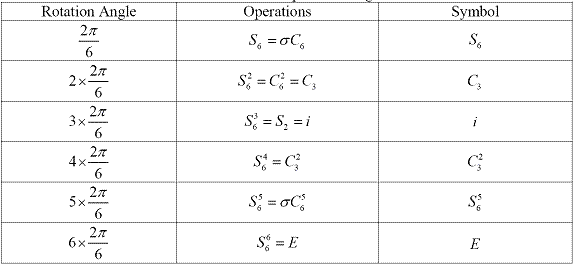Table 1.4 S5 axis and operations it generates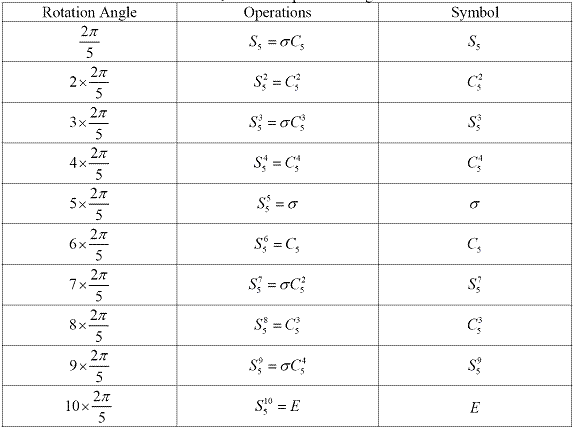Figure 1.5 Improper rotation operation S4 in CH4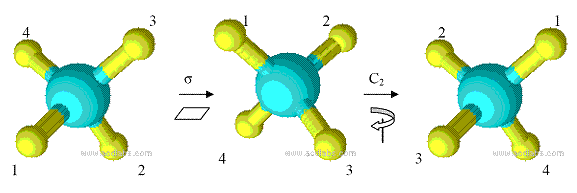## Symmetry Point Groups

For a molecule, all the symmetry operations that can be applied to the molecule have all the properties of a group. Therefore, before we introduce the symmetry point groups, the concept and properties of a group will be introduced first. When some elements have a certain kind of relationships and can be related to each other by these relationships, these elements can form a group.

#### Closure

If two elements A and B are in the group G, then the multiplicity of these two elements, C, is also in this group. It can be expressed as

#### Associativity

All the elements in the group must satisfy the law of associativity, which can be expressed as

(AB)C=A(BC)

#### Identity

The group must contain such an element E that

ER=RE=R

In group theory, it refers to the operation identity E. Because any molecule or substance must at least have the symmetry element E.

#### Inverses

If A is an element in group G, there must be another element A-1 in group G that satisfies AA-1= A-1A=E. Usually we can write A-1 as B. It can be expressed as

If A?G and AA-1= A-1A=E then A-1=B=G

## Group Multiplication Tables

If there are n elements in a group G, and all of the possible n2 multiplications of these elements are known, then this group G is unique and we can write all these n2 multiplications in a table called group multiplication table. All the symmetry operations of a molecule can be written in the form of group multiplication table. There is a very important rule about group multiplication tables called rearrangement theorem, which is that every element will only appear once in each row or column.1In group theory, when the column element is A and row element is B, then the corresponding multiplication is AB, which means B operation is performed first, and then operation A follows.1

Table 2.1 Group multiplication table1

 G6 E A B C D F E E A B C D F A A E D F B C B B F E D C A C C D F E A B D D C A B F E F F B C A E D

## Subgroups

From table 2.1 above, we can find several small groups in the group multiplication table. For example,

 G2 E A E E A A A E

In the same way, there are also many several small groups with orders 1, 2, 3 respectively. These small order groups that can be found in a higher order group are called subgroups. The number of elements in a group is called the order of a group, using a symbol h. The number of elements consist a subgroup is called the order of a subgroup, using a symbol g. From the previous two examples, we have1,

h/g=k (k is a whole number)

Since the symmetry point group have all the properties of a group, there are also several subgroups that we can find in a perticular symmetry point group. And sometimes we just use symmetry opertaions in one subgroup to apply to a system instead of using all the symmetry operations in the group, which can significantly simplify the calculations.

## Classes

Class is another important concept in group theory which provides a way to simplify the expression of all the symmetry operations in a group. This means that we do not have to write down all the symmetry operatoins in a group but combine some related operations instead. The followin part will introduce the concept of classes and how to divide a group into classes.

### Similarity transformation and conjugate:

A and B are two elements in a group, X is any elements in this group. If

$X^{-1}AX=B$

Then we can say the relationship of A and B is similarity transformation. A and B are conjugate.1 Conjugate elements have three properties1:

1. Every element is conjugate with itself. $A=X^{-1}AX$
2. If A is conjugate with B, then B is conjugate with A. $\text{If} A=X^{-1}BX, \text{then} B=Y^{-1}AY$
3. If A is conjugate with B and C, then B is conjugate with C. $\text{If} A=X^{-1}BX \text{ and } A=Y^{-1}CY, \text{then} B=Z^{-1}CZ$

### Classes

Now we can define a class. A class of a group is defined as all the elements in the group that are conjugated to each other.1 To determine the classes of a group, we need to apply similarity transformation to the elements in the group until all the elements are grouped into smaller sets. For example, there are four elements in a group: {E, C2, σv, σv}

X= E, C2, $$\sigma_v$$, $$\sigma_v$$ X-1= E, C2, σv, σv

Because we always have XEX-1=E, {E} is always a class for any point group. Then, apply similarity transformation to other elements in the group until all the elements are classified in smaller sets. Table 2.3 shows how the elements are classified in to classes.

Table 2.4 Classes six order groupTherefore, there are three classes in group C3v: {E}, {C3, C32} and { σv, σv , σv '‘ }.

Significance of classes of a group

In the same class of a group, the operations can be converted to each other by an operation. The operations in the same class are called equivalent operations. And a class of a symmetry group is a group of equivalent operations. This gives a simpler way to express the operations in a group.1For example, for operations in the six order group above, table 2.5 shows the new way to express these operations.

Table 2.5 Ways to express operations in six order group## Symmetry Point Groups

As what mentioned above, all the symmetry operations of a molecule as a group can be written in the form of group multiplication table and they obey all the properties of a group. This group is called symmetry point group. It is called point group for two reasons. First reason is that this group have all the properties of a group. Second reason is that all the symmetry operations are related to a fixed point in the molecule, which is not necessarily to be an atom of the molecule. According to the symmetry of molecules, they can be classified as symmetry point groups.1To determine the symmetry point group of a molecule is very important, because all symmetry related properties are dependent on the symmetry point group of the molecule. Symmetry point groups can be divided into 5 classes which are summarized below and the they are described in details here (symmetry point groups).1,6

Point groups Symmetry Elements Order Example
Nonaxial C1 E 1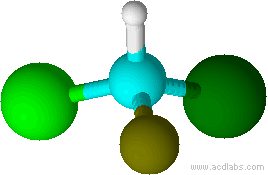HCFBrCl
Ci E, i 2C2H2F2Cl2
Cs E, ? 2CH2BrCl
Cyclic Cn E, Cn nC2H4Cl2
Cnh E, Cn, n$$\sigma_v$$ 2nNH3
Cnv E, Cn, σh, Sn 2nC2H2F2
Sn E, Sn n1,3,5,7 -tetrafluoracyclooctatetrane
Dihedral Dn E, Cn, nC2 (?Cn) 2n[Co(en)3]3+.
Dnh E, Cn, σh, nC2 (?Cn) 4nBenzene

Dnd E, Cn, σd, nC2 (?Cn) 4nC2H6
Polyhedral Td E, 3C2, 4C3, 3S4, d 24CCl4
Oh E, 3S4, 3C4, 6C2, 4S6, 4C3, 3σh, 6σd, i 48SF6
Ih E, 6S10, 10S6, 6C5, 10C3, 15C2, 15? 120C60
Linear C$$\sigma_v$$ E, C?, σv ?HCl
D$$\sigma_h$$ E, C? σv , σh, i, ?C2 ?O2

## Determination of symmetry Point Groups

Determination of symmetry point group of a molecule is the very first step when we are solving chemistry problems. The symmetry point group of a molecule can be determined by the following flow chart7.

Table 2.12 Flow chart to determine point groupNow, using this flow chart, we can determine the symmetry of molecules. However, to further determine the symmetry properties of something such as molecular orbitals, vibrational modes, etc. we need character tables which will be introduced next.

## Representations of a Group

Through similarity of transformation, we can define the reducible and irreducible representations of a group. If a matrix representation A can be transferred to block-factored matrix A’, a matrix composed of blocks (A’, A’’, A’’’) at the diagonal and zero in any other position, by similarity transformation, this matrix A is called the reducible representation of this group. And if these blocks (A’, A’’, A’’’) cannot be further transferred to block-factored matrix through similarity transformation, A’, A’’, A’’’ are called irreducible representations of the group. And the sum of the trace of A’, A’’, A’’’ (the number on the diagonal of A’, A’’, A’’’) is called the characters of this representation. As is shown in the following equation, a11’+ a22’+…+ ann’ is one of the characters. Reducible representations can be reduced to irreducible representations and irreducible representations cannot be reduced further.1Take C3v as an example.Take (x, y, z) as the basic, the matrix of all the operations in C3v group {E, C3, C32, σv, σv , σv '‘} are shown in Table 2.6.

Table 2.6 Reducible and irreducible representations of C3v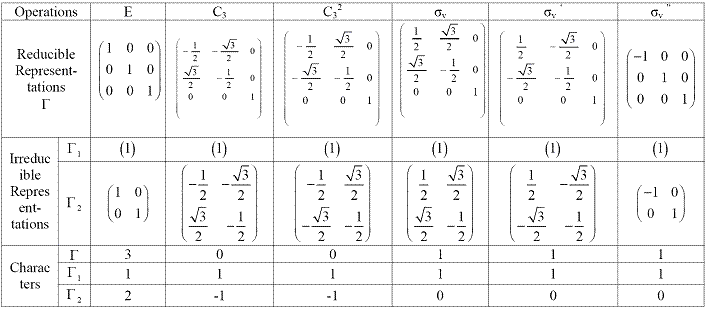As shown in the table 2.6, all the matrix are block-factored matrix and they are reducible representations. Every block in these reducible representations are irreducible representations and the sums of the trace are the characters which are also listed in the table. Notice that the operations in the same class have the same character. Therefore, they are always written together using the new notations {E, 2C3, 3σv}:

Table 2.7 Two irreducible representations of C3v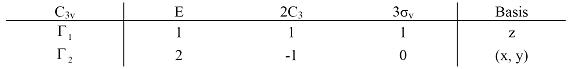This is not a completed table of irreducible representations, because the basis chosen are not completed. There are different kinds of basis, including one-dimensional basis like x, y, z, Rx, Ry, Rz and two-dimensional basis like x2, y2, z2, xy, yz, xz. If we chose Rz which is the rotation around z-axis as another basis, we can get another irreducible representation, (1, 1, -1). “1” means nothing change when operation is applied to the basis. “-1” means it becomes opposite when operation is applied to the basis. “0” means the basis are moved when operation is applied. Therefore, in summary, for now the irreducible representations of C3v group is

Table 2.8 Summary of irreducible representations of C3v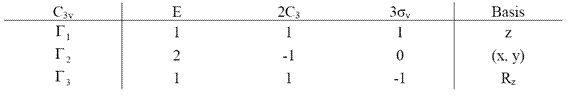## Five rules of Characters

For irreducible representations and their characters, there are five very important rules1:

### Rule 1

The order of the irreducible representation matrix is called the dimension of the irreducible representation, using symbol l1, l2, ... And rule 1 states that the sum of the squares of all the dimensions of irreducible representations (l12+ l22+ …) is equal to the order of the group, h.

?li2=l12+ l22+ …=h

Since the character of the irreducible representation of operation E, ?i(E), is equal to the dimension of the corresponding irreducible representation, Rule 1 can also be written as

? Γi(E)2= Γ1(E)2+ Γ2(E)2+ …=h

### Rule 2

Rule 2 is that the sum of the square of the characters in any irreducible representation is equal to h. For example, for the first irreducible representation in C3v group

Γ1(E)2+ Γ1(C3)2+ Γ1(C32)2+ Γ1v)2+ Γ1v )2+ Γ1v '‘)2=12+12+12+12+12+12=6

Since for the same class, the characters are the same, it can also be written as

Γ1(E)2+ 2*Γ1(C3)2+ 3* Γ1v)2=12+2*12+3*12=6

Also, for another irreducible representation in C3v group

Γ1(E)2+ 2*Γ1(C3)2+ 3* Γ1v)2=22+2*(-1)2+3*02=6

### Rule 3

Rule 3 is that the vectors which composed of the characters from different irreducible representations are orthogonal.

? ?i(R)2Γj(R)2 =0 (i is not equal to j)

Again, take C3v group for an example

? Γ1(R)2Γ2(R)2 =1*2+2*1*(-1)+3*1*0=0

### Rule 4

Rule 4 is that the characters of matrix representations, either reducible or irreducible, of the operations in the same class are the same, which is shown as the former table.

### Rule 5

Rule 5 is that the number of irreducible representations is equal to the number of classes.

For C3v group, there are three classes and therefore there are also three irreducible representations. Therefore, the three irreducible representations in Table 2.8 are the complete list of irreducible representations in C3v group.

There is a very important relationship between reducible representations and irreducible representations, which is that any reducible representation can be written as the linear combination of irreducible representations. The similarity transformations do not change the character of a reducible representation, thereforewhere ?(R) is the character of reducible representation of operation R. ?j(R) is the character of jth irreducible representation. aj is the times ?j(R) will appear in blocks when reducible representation is reduced to irreducible representation by similarity transformation. To determine aj, the former equation can be written asAccording to rule 3, only when i=j, the sum over R is not equal to zero. Then according to rule 1, the equation can be written asThen rearrange toUsing this equation, we can express reducible representations with irreducible representations, which is very important when we solve chemistry problems.

Again, take C3v group for an example, the reducible representation shown in table 2.6 can be express as combination of irreducible representations using this relationship. Use irreducible representations in table 2.8

a1= 1/6 [1(3)(1)+2(0)(1)+3(1)(1)] = 1

a1= 1/6 [1(3)(2)+2(0)(-1)+3(1)(0)] = 1

a1= 1/6 [1(3)(1)+2(0)(1)+3(1)(-1)] = 0

Therefore,5

Table 2.11 Meanings of different area of character tables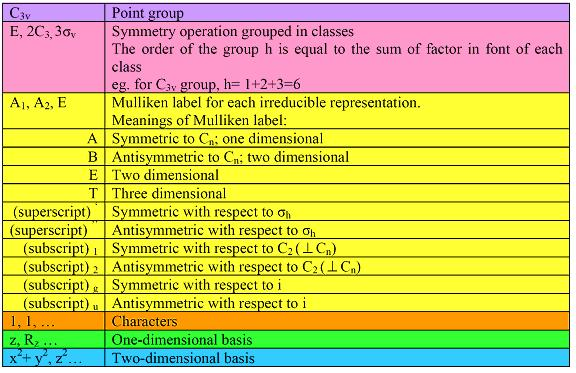Using this flow chart, you can determine the symmetries of small molecules. And also, by using group theory and character tables, you can determine the symmetries of any thing you are interested in, such as molecular orbitals, vibrational modes, etc. In conclusion, group theory play a very important role in chemistry, which we can see from various applications of group theory in chemistry, like Infrared spectrum, Raman spectrum, electronic spectrum, etc.